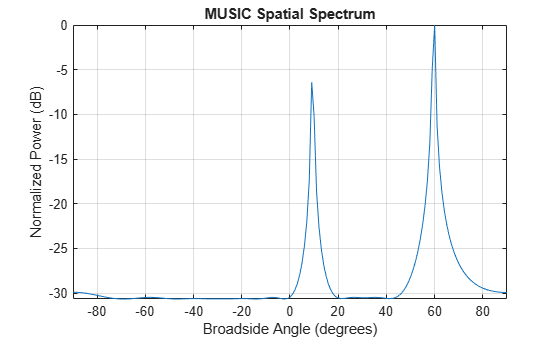# plotSpectrum

System object: phased.MUSICEstimator
Package: phased

Plot MUSIC spectrum

## Syntax

```plotSpectrum(estimator) output_args = plotSpectrum(estimator,Name,Value) lh = plotSpectrum(___) ```

## Description

`plotSpectrum(estimator)` plots the MUSIC spectrum computed by the most recent `step` method execution for the `phased.MUSICEstimator` System object™, `estimator`.

`output_args = plotSpectrum(estimator,Name,Value)` plots the MUSIC spatial spectrum with additional options specified by one or more `Name,Value` pair arguments.

`lh = plotSpectrum(___)` returns the line handle to the figure.

## Input Arguments

expand all

MUSIC estimator, specified as a `phased.MUSICEstimator` System object

### Name-Value Arguments

Specify optional pairs of arguments as `Name1=Value1,...,NameN=ValueN`, where `Name` is the argument name and `Value` is the corresponding value. Name-value arguments must appear after other arguments, but the order of the pairs does not matter.

Before R2021a, use commas to separate each name and value, and enclose `Name` in quotes.

Units used for plotting, specified as the comma-separated pair consisting of `'Unit'` and `'db'`, `'mag'`, or `'pow'`.

Data Types: `char`

Plot a normalized spectrum, specified as the comma-separated pair consisting of `'NormalizedResponse'` and `false` or `true`. Normalization sets the magnitude of the largest spectrum value to one.

Data Types: `char`

Title of plot, specified as a comma-separated pair consisting of `'Title'` and a character vector.

Example: `true`

Data Types: `char`

## Output Arguments

expand all

Line handle of plot.

## Examples

expand all

Estimate the DOAs of two signals received by a standard 10-element ULA having an element spacing of 1 meter. Then plot the MUSIC spectrum.

Note: You can replace each call to the function with the equivalent `step` syntax. For example, replace `myObject(x)` with `step(myObject,x)`.

Create the ULA array. The antenna operating frequency is 150 MHz.

```fc = 150.0e6; array = phased.ULA('NumElements',10,'ElementSpacing',1.0);```

Create the arriving signals at the ULA. The true direction of arrival of the first signal is 10° in azimuth and 20° in elevation. The direction of the second signal is 60° in azimuth and -5° in elevation.

```fs = 8000.0; t = (0:1/fs:1).'; sig1 = cos(2*pi*t*300.0); sig2 = cos(2*pi*t*400.0); sig = collectPlaneWave(array,[sig1 sig2],[10 20; 60 -5]',fc); noise = 0.1*(randn(size(sig)) + 1i*randn(size(sig)));```

Estimate the DOAs.

```estimator = phased.MUSICEstimator('SensorArray',array,... 'OperatingFrequency',fc,... 'DOAOutputPort',true,'NumSignalsSource','Property',... 'NumSignals',2); [y,doas] = estimator(sig + noise); doas = broadside2az(sort(doas),[20 -5])```
```doas = 1×2 9.5829 60.3813 ```

Plot the MUSIC spectrum.

`plotSpectrum(estimator,'NormalizeResponse',true)`## Version History

Introduced in R2016b